Courses

# 28 Year NEET Questions: Waves- 2

## 30 Questions MCQ Test Physics Class 11 | 28 Year NEET Questions: Waves- 2

Description
This mock test of 28 Year NEET Questions: Waves- 2 for NEET helps you for every NEET entrance exam. This contains 30 Multiple Choice Questions for NEET 28 Year NEET Questions: Waves- 2 (mcq) to study with solutions a complete question bank. The solved questions answers in this 28 Year NEET Questions: Waves- 2 quiz give you a good mix of easy questions and tough questions. NEET students definitely take this 28 Year NEET Questions: Waves- 2 exercise for a better result in the exam. You can find other 28 Year NEET Questions: Waves- 2 extra questions, long questions & short questions for NEET on EduRev as well by searching above.
QUESTION: 1

### A cylindrical resonance tube open at both ends, has a fundamental frequency, f, in air. If half of the length is dipped vertically in water, the fundamental frequency of the air column will be

Solution:

Fundamental frequency of open pipe,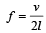When half of tube is filled with water, then the length of air column becomes half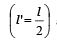and the pipe becomes closed.

So,  new fundamental frequency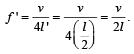Clearly f ' = f.

QUESTION: 2

### A transverse wave is represented by the equation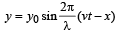For what value of λ is the maximum particle velocity equal to two times the wave velocity? 

Solution: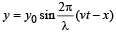Particle velocity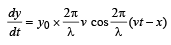Maximum particle velocity =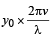Wave velocity = v [given]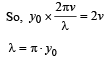QUESTION: 3

### A vehicle, with a horn of frequency n is moving with a velocity of 30 m/s in a direction perpendicular to the straight line joining the observer and the vehicle. The observer perceives the sound to have a frequency n + n1.Then (if the sound velocity in air is 300 m/s) 

Solution:

As the source is not moving towards or away from the observer in a straight line, so the Doppler’s effect will not be observed by the observer.

QUESTION: 4

A standing wave having 3 nodes and 2 antinodes is formed between two atoms having a distance 1.21 Å between them. The wavelength of the standing wave is 

Solution:

Let  be length of string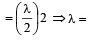Hence, the wave length of standing wave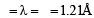QUESTION: 5

In a sinusoidal wave, the time required for a particular point to move from maximum displacement to zero displacement is 0.170 sec.The frequency of the wave is 

Solution:

Time taken to move from maximum to zero displacement =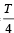∴ Time period T = 4 × 0.170 second

∴  Frequency,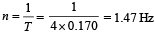QUESTION: 6

Resonance is an example of 

Solution:

We know that if frequency of an external forced  oscillation is equal to the natural frequency of the body, then amplitude of the forced oscillation of the body becomes very large. This phenomenon is known as resonant vibration. Therefore, resonance is an example of forced vibration.

QUESTION: 7

Two waves of lengths 50 cm and 51 cm produce 12 beats per sec. The velocity of sound is  

Solution:

Given : Wavelength of first wave (λ1) = 50 cm = 0.5 m Wavelength of second wave (λ2) = 51 cm = 0.51m

frequency of beats per sec (n) = 12.
We know that the frequency of beats,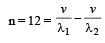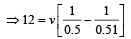= v[2 - 1.9608]  = v x 0.0392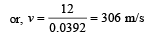[where, v = velocity of sound]

QUESTION: 8

If n1, n2 and n3 are the fundamental frequencies of three segments into which a string is divided, then the original fundamental frequency n of the string is given by 

Solution: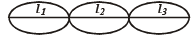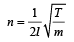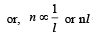= constant, K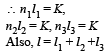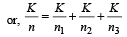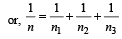QUESTION: 9

Two sound sources emitting sound each of wavelength λ are fixed at a given distance apart.
A listener moves with a velocity u along the line joining the two sources. The number of beats heard by him per second is 

Solution:

Frequency received by listener from the rear source,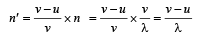Frequency received by listener from the front source,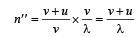No. of beats = n'' – n'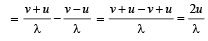QUESTION: 10

The equation of a wave is represented by: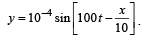The velocity of the wave will be 

Solution: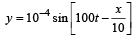v = 100 sec–1, λ = 10 m

v = λv = 10 × 100 = 1000 m/s

QUESTION: 11

A source and an observer move away from each other, with a velocity of 10m/s with respect to ground. If the observer finds the frequency of sound coming from the source as 1950 Hz, then original frequency of source is (velocity of sound in air = 340 m/s) 

Solution:

According to Doppler's effect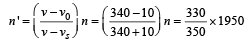= 2068 Hz

QUESTION: 12

A string of 7 m length has a mass of 0.035 kg. If tension in the string is 60.5 N, then speed of a wave on the string is 

Solution:

Given : Length (l) = 7 m
Mass (M) = 0.035 kg and tension (T) = 60.5 N.
Therefore, mass of string per unit length (m)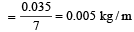speed of wave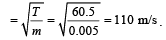QUESTION: 13

The equation for a transverse wave travelling along the positive x-axis with amplitude 0.2 m, velocity v =360 ms–1 and wavelength λ = 60 m can be written as 

Solution:

v = nλ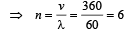a = 0.2

For a wave travelling along positive x-axis y  = a sin (ωt – kx)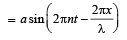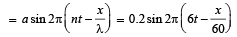QUESTION: 14

A whistle of frequen cy 385 Hz rotates in a horizontal circle of radius 50 cm at an angular speed of 20 radians s–1. The lowest frequency heard by a listener a long distance away at rest with respect to the centre of the circle, given velocity of sound equal to 340 ms–1, is 

Solution:

Velocity of source

vs = rω =  0.50 x 20 = 10 ms-1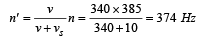QUESTION: 15

An observer moves towards a stationary source of sound with a speed 1/5th of the speed of sound. The wavelength and frequency of the sound emitted are λ and f respectively. The apparent frequency and wavelength recorded by the observer are respectively. 

Solution: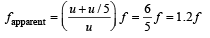Wavelength remains constant (unchanged) in this case

QUESTION: 16

A car is moving towards a high cliff. The car driver sounds a horn of frequency f. The reflected sound heard by the driver has frequency 2f. If v be the velocity of sound, then the velocity of the car, in the same velocity units, will be

Solution:

Let f ' be the frequency of sound heard by cliff.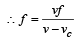....(1)

Now, for the reflected wave, cliff acts as a source,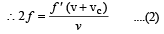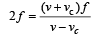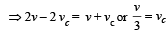QUESTION: 17

The phase difference between two waves, represented by 
y1 = 10–6 sin{100 t + (x/50) + 0.5} m
y2 = 10–6 cos{100 t + (x/50)} m
where x is expressed in metres and t is expressed in seconds, is approximately 

Solution:

y1 = 10–6 sin (100t + x/50 + 0.5)m
= 10–6 cos (100t + x/50 – π/2 + 0.5)m
y2  = 10–6 cos (100t + x/50)m

∴ φ = π/2 – 0.5 = 1.07 rad

QUESTION: 18

Two vibrating tuning for ks produce progressive waves given by y1 = 4 sin 500 πt and y2 = 2 sin 506 πt. Number of beats produced per minute is [2 00 5]

Solution:

Equation of progressive wave is given by y = A sin2πft
Given y1 = 4sin500 πt and y2 = 2sin506πt.
Comparing the given equations with equation of progressive wave, we get 2f1 = 500 ⇒  f1 = 250 2f2 = 506 ⇒ f2 = 253
Beats = f2– f1 = 253 – 250 = 3 beats/sec = 3 × 60 = 180 beats/minute.

QUESTION: 19

A point source emits sound equally in all directions in a non–absorbing medium. Two points P and Q are at distances of 2m and 3m respectively from the source. The ratio of the intensities of the waves at P and Q is 

Solution:

Intensity = Energy/sec/unit area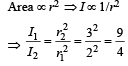QUESTION: 20

Which one of the following statements is true ? 

Solution:

Sound waves in air are longitudinal and the light waves are transverse.

QUESTION: 21

A transverse wave propagating along x-axis is represented by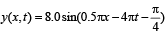where x is in metres and t is in seconds. The speed of the wave is 

Solution:

Speed of a wave represented by the equation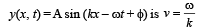By comparison, ω = 4π; k = 0.5π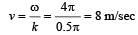QUESTION: 22

The time of reverberation of a room A is one second. What will be the time (in seconds) of reverberation of a room, having all the dimensions double of those of room A ?

Solution:

Reverberation time is defined as the time during which the intensity of sound in the auditorium becomes one millionth of the initial intensity.
Sabine has shown that standard reverberation time for an auditorium is given by the formula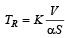Here, V is volume of the auditorium, S is the surface area. So,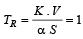(given)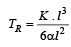(Assuming auditorium to be cubic in shape)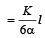So, TR α l

If dimension is doubled, reverberation time t will be doubled. So, New TR = 2 sec.

QUESTION: 23

Two sound waves with wavelengths 5.0 m and 5.5m respectively, each propagate in a gas with velocity 330 m/s. We expect the following number of beats per second 

Solution:

Frequencies of sound waves are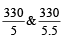i.e.,  66 Hz and 60 Hz
Frequencies of beat = 66 – 60 = 6 per second

QUESTION: 24

Two points are located at a distance of 10 m and 15 m from the source of oscillation. The period of oscillation is 0.05 sec and the velocity of the wave is 300 m/sec. What is the phase difference between the oscillations of two points? 

Solution:

Here, T = 0.05 sec, v = 300 ms–1.
Now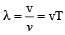= (300 x 0.05)m

or, λ = 15 m

Phase of the point at 10 m from the source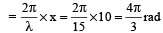Phase of the point at 15 m from the source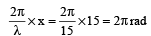∴ The phase difference between the points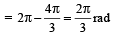QUESTION: 25

The wave described by y = 0.25 sin (10πx – 2πt), where x and y are in meters and t in seconds, is a wave travelling along the: 

Solution:

y = 0.25 sin (10πx – 2πt)

Comparing this equation with the standard wave equation y = asin (kx – ωt)

We get,  k = 10π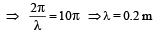And ω = 2π or, 2πv = 2π ⇒ v = 1Hz.
The sign inside the bracket is negative, hence the wave travels in + ve x- direction.

QUESTION: 26

Each of the two strings of length 51.6 cm and 49.1 cm are tensioned separately by 20 N force.Mass per unit length of both the strings is same and equal to 1 g/m. When both the strings vibrate simultaneously the number of beats is

Solution:

The frequency of vibration of a string is
given by,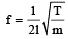where m is mass per unit length.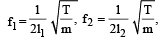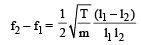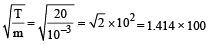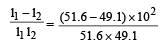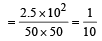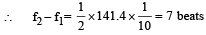QUESTION: 27

The driver of a car travelling with speed 30 m/sec towards a hill sounds a horn of frequency 600 Hz. If the velocity of sound in air is 330 m/s, the frequency of reflected sound as heard by driver is 

Solution: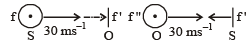f' is the apparent frequency received by an observer at the hill. f'' is the frequency of the reflected sound as heard by driver.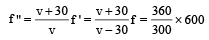= 720 Hz

QUESTION: 28

A wave in a string has an amplitude of 2 cm. The wave travels in the + ve direction of  x axis with a speed of 128 m/sec and it is noted that 5 complete waves fit in 4 m length of the string.The equation describing the wave is 

Solution: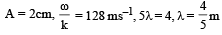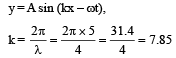y = 0.02 m sin (7.857 – 1005 t)

ω  = 128 × 7.85 = 1005

QUESTION: 29

A transverse wave is represented by y = A sin (ωt–kx). For what value of the wavelength is the wave velocity equal to the maximum particle velocity? 

Solution:

y = A sin (ωt–kx)

Particle velocity,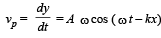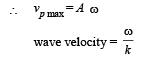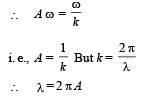QUESTION: 30

A tuning fork of freqqency 512 Hz makes 4 beats per second with the vibrating string of a piano.The beat frequency decreases to 2 beats per sec when the tension in the piano string is slightly increased. The frequency of the piano string before increasing the tension was 

Solution:

The frequency of the pianostring = 512 ± 4 = 516 or 508. When the tension is increased, beat frequency decreases to 2, it means that frequency of the string is 508 as frequency of string increases with tension.